Courses

# Test: Quantitative Techniques- 2

## 20 Questions MCQ Test Mock Test Series for CLAT 2021 | Test: Quantitative Techniques- 2

Description
This mock test of Test: Quantitative Techniques- 2 for Banking Exams helps you for every Banking Exams entrance exam. This contains 20 Multiple Choice Questions for Banking Exams Test: Quantitative Techniques- 2 (mcq) to study with solutions a complete question bank. The solved questions answers in this Test: Quantitative Techniques- 2 quiz give you a good mix of easy questions and tough questions. Banking Exams students definitely take this Test: Quantitative Techniques- 2 exercise for a better result in the exam. You can find other Test: Quantitative Techniques- 2 extra questions, long questions & short questions for Banking Exams on EduRev as well by searching above.
QUESTION: 1

### In a class average age of 24 boys and their teacher is 15 years. When the teacher's age is excluded the average age decreases by one year. The age of the teacher is?

Solution:

Sum of the ages of 24 boys and 1 teacher = (15 x 25) years = 375 years.
Sum of the ages of 24 boys = (24 x 14) years = 336 years.
Age of the teacher = (375 − 336) years = 39 years.

QUESTION: 2

### The monthly income of P and Q are in ratio 2:3 and their monthly expenses are in ratio 5:9. If each of them save 300 per month, their monthly income is:

Solution:

Income of A = 2x
Income of B = 3x

Savings of A  =  300
Savings of B  =  300

Monthly expense are in ratio 5:9
(2x-300) / (3x-300) =  5/9
⇒ 18x - 2700 = 15x - 1500
⇒ 3x = 1200
⇒ x =  400

Therefore,
Income of A = 2x = 2 * 400 = 800
Income of B = 3x = 3 * 400 = 1200

QUESTION: 3

### Man covers a certain distance by driving a car at the speed of 60 km/h and return back to the starting point at the speed of 40 km/h. Find its average speed?

Solution: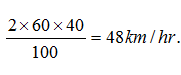QUESTION: 4

Two numbers are such that the ratio between them is 3:4 but if each is increased by 3 then the ratio becomes 4:5. Find the difference between the numbers?

Solution:

Let the two numbers be 3x, 4x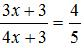⇒ 15x + 15 = 16x + 12
⇒ x = 3
Diff = (4*3) - (3*3) = 3

QUESTION: 5

At present, the age of the father is five times that the age of the son. Three years hence, the father's age would be four times that of his son. Find the present age of the father and the son?

Solution:

Let the son's age be x.
⇒ Father's age = 5x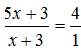⇒ 5x + 3 = 4x + 12
⇒ x = 9
Hence, son's age is 9 and father's age is 45.

QUESTION: 6

If 25% of a number is 65, then 45% of the number will be?

Solution: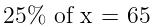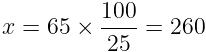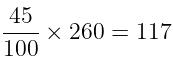QUESTION: 7

A and B have money in the ratio 2:1. If A gives Rs. 2 to B, the money will be in the ratio 1:1. What were the initial amounts they had?

Solution:

Before lending the money, the ratio of money A and B = 2:1
After lending the money, the ratio of money A and B = 1:1
Since, A gives B two rupees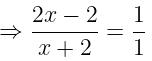⇒ 2x - 2 = x + 2
⇒ x = 4

A = 2 × 4 = 8, B = 1 × 4 = 4

QUESTION: 8

18 men can reap a field in 50 days. In how many days will 30 men can complete the same work?

Solution:

18 * 50 = Days * 30
⇒ Days = 30

QUESTION: 9

A is working and B is sleeping partner in a firm. A invest Rs 2100 and B invests Rs 3100. A being working partner gets 25% of the profits, and the balance of the profits to be divided in the proportion to their capitals. What did each get out of profit of Rs 1040?

Solution:

Total profit = 1040.
A's 25% profit = 1040 * 1/4 = 260
Remaining profit = 780
Ratio of investment of A and B = 2100:3100 = 21:31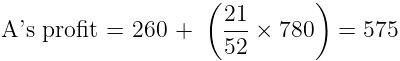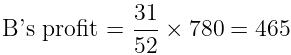QUESTION: 10

A man has some hens and cows. If the number of heads be 48 and the number of feet equals 140, then the number of hens will be:

Solution:

Let the number of hens be x and the number of cows be y.
Then, x + y = 48 .... (i)
and 2x + 4y = 140
⇒  x + 2y = 70 .... (ii)
Solving (i) and (ii) we get: x = 26, y = 22.
∴ The required answer = 26.

QUESTION: 11

If a man can row at the rate of 5kmph instill water and his rate against current is 3.5kmph, then man's rate of speed along current is?

Solution:

Speed of Boat = 5 Km/h
Speed upstream = 3.5 km/h

Speed of upstream = Speed of boat - Speed of water

⇒ Speed of water = 5 - 3.5 = 1.5 km/h
⇒ Speed downstream = 5 + 1.5 = 6.5 km/h

QUESTION: 12

If a square field has its area equal to 324m2 then the perimeter of the field?

Solution:

Area = 342 m2
⇒ Side = √342 = 18m
⇒ Perimeter = 18 x 4 = 72m

QUESTION: 13

X alone can complete a piece of work in 40 days. He worked for 8 days and left. Y alone complete the remaining work in 16 days. How long would X and Y together take to complete the work?

Solution: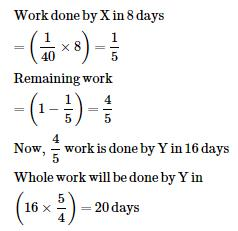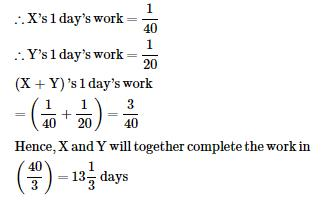QUESTION: 14

The difference between simple interest and compound interest on certain sum of money for 2 years 4% per annum is Rs 1. Find the sum?

Solution: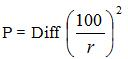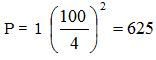QUESTION: 15

By selling a bicycle for Rs. 2,850, a shopkeeper gains 14%. If the profit is reduced to 8%, then the selling price will be:

Solution:

Let Cost Price was X.
X + 14% of X = 2850
X + 0.14X = 2850
1.14X = 2850
X = 2500.
⇒ Cost Price = Rs. 2500.
Now, Selling Price when profit remains at 8% = 2500 + 8% of 2500 = Rs. 2700

QUESTION: 16

A men went to RBI with Rs 2000. He asked the cashier to give him Rs 10 and Rs 20 notes only in return. The man got 150 notes in all. How many Rs 10 notes did he get in all?

Solution:

x + y = 150 ........(i)
10x + 20y = 2000
⇒  x + 2y = 200 .......(ii)
Solving (i) and (ii),we get
y = 50, x = 100

QUESTION: 17

If price of a book is first decreased by 25% and then increased by 20% the net percentage change in the price of the book will be

Solution:

Let the original price be Rs. 100.

New final price = 120% of (75% of Rs. 100) = 0.12 * (0.75 * 100) = Rs. 90.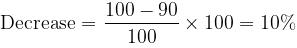QUESTION: 18

A dice is rolled twice. What is probability of getting the sum 5?

Solution:

Sum of 5 is obtained as (1,4), (2,3), (3,2), (4,1)
⇒ Probability = 4/36 = 1/9

QUESTION: 19

12345 + 13000 + 41240 + 8 = ?

Solution:

12345 + 13000 + 41240 + 8 = 66593

QUESTION: 20

Three numbers are in the ratio  1 : 2 : 3  and their H.C.F is 12. The numbers are

Solution:

Let the required numbers be x, 2x, 3x.
Since H.C.F. = 12
⇒ The numbers are: 12, 24, 36